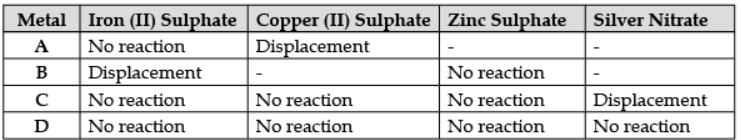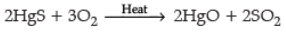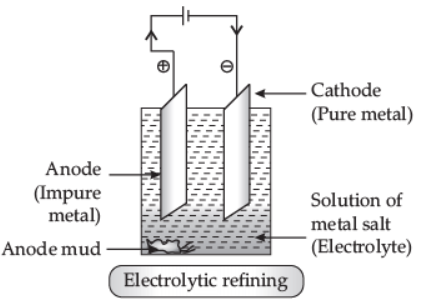Courses

# Worksheet (1): Metals and Non-metals Class 10 Notes | EduRev

## Class 10 : Worksheet (1): Metals and Non-metals Class 10 Notes | EduRev

The document Worksheet (1): Metals and Non-metals Class 10 Notes | EduRev is a part of the Class 10 Course Science Class 10.
All you need of Class 10 at this link: Class 10

Q.1. Which one of the following metals does not react with cold as well as hot water?
(a)
Na
(b) Ca
(c) Mg
(d) Fe
Ans. (d)
Solution. Metals like aluminium, zinc, iron do not react with hot/cold water.
3Fe + 4H2O → Fe3O4 + 4H2.

Q.2. Study the given table and answer the following questions:
A student took the samples of four metals A, B, C and D and added the following solution one by one. The results obtained have been tabulated as follows:(a) Which is the most reactive metal? (1 Marks)
(b) What would you observe if B is added to a solution of Copper (ii) Sulphate? (1 Marks)

(c) Arrange the metals A, B, C and D in the order of decreasing reactivity? (1 Marks)(d) Which gas is produced when dilute HCl is added to a reactive metal? (1 Marks)
Ans.
(a) B is the most reactive metal as it displaces iron from its salt solution.
(b) B will displace Cu from CuSO4 solution because B is more reactive than copper.
(c) B > A > C > D
(d) Hydrogen gas is produced when dilute HCl is added to a reactive metal.

Q.3. What is meant by metallurgy? (1 Marks)
Ans.
The extraction of metals from their ores and then refining them for use is known as metallurgy.

Q.4. (a) Write chemical equations for the following reactions :  (5 Marks)
(i) Calcium metal reacts with water.
(ii) Cinnabar is heated in the presence of air.
(iii) Manganese dioxide is heated with aluminium powder.
(b) What are alloys? List two properties of alloys.
Ans.
(a) (i) Ca + 2H2O → Ca(OH)2 + H2
(ii)(iii) 3MnO2 + 4Al → 2Al2O3 + 3Mn
(b) Alloys are homogeneous mixtures of two or more metals or a metal and a non-metal.
Properties: Alloys are stronger / harder / have low melting point / more resistant to corrosion / some are magnetic in nature. (Any two)

Q.5. Describe electrolytic refining of copper with chemical equations. Draw a well-labelled diagram for it.  (3 Marks)
Ans.
Electrolytic Refining: This method is widely used as purification of metals like zinc (Zn), copper (Cu), aluminium (Al), chromium (Cr), tin (Sn), lead (Pb), nickel (Ni) and gold (Au). In this process, impure metal is used as anode, a strip of pure metal is used as cathode, and soluble salt of metal is used as electrolyte. On passing electric current through the electrolyte, cations move towards the cathode, gain electrons, and pure metal gets deposited on the cathode. In electrolytic refining of copper, the impurities left behind at anode called anode mud contain valuable metals such as gold and silver, which can be recovered in the native state.Q.6. Name a non-metal which is lustrous and a metal which is non-lustrous. (1 Marks)
Ans.

Non-metal (lustrous) = Iodine (I)
Metal (non-lustrous) = Sodium (Na)

Q.7. Assertion and Reason :
Directions: In the following questions, a statement of assertion (A) is followed by a statement of reason (R). Mark the correct choice as :

(a) Both assertion (A) and reason (R) are true and reason (R) is the correct explanation of assertion (A).
(b) Both assertion (A) and reason (R) are true but reason (R) is not the correct explanation of assertion (A).
(c) Assertion ( A) is true but reason (R) is false.
(d) Assertion ( A) is false but reason (R) is true.
(i) Assertion (A): When a piece of copper metal is added to dilute sulphuric acid, the solution turns blue.
Reason (R): Copper reacts with dilute sulphuric acid to form blue copper (ii) sulphate solution.  (1 Marks)
Ans. (a)
Solution. When a piece of copper metal is added to dilute sulphuric acid, the solution turns blue. It is because copper reacts with dilute sulphuric acid to form blue copper (II) sulphate solution.

(ii) Assertion (A): Metals are sonorous.
Reason (R): They are generally brittle in the solid-state; they break into pieces when hammered.  (1 Marks)
Ans. (c)
Solution. Metals are sonorous, malleable and ductile while non-metals are brittle.

(iii) Assertion (A): Gas bubbles are observed when sodium carbonate is added to dilute hydrochloric acid.
Reason (R): Carbon dioxide is given off in the reaction.  (1 Marks)
Ans. (a)
Solution. Gas bubbles are observed when sodium carbonate is added to dilute hydrochloric acid as CO2 gas is released.

Q.8. What happens when zinc granules are treated with dilute solutions of H2SO4, HCl, HNO3, NaCl and NaOH? Also, write the chemical equation. (5 Marks)
Ans. (i) With dilute H2SO4: H2 gas is evolved.
Zn + H2SO4 → ZnSO4 + H2
(ii) With dilute HCl: H2 gas is evolved.
Zn + 2HCl → ZnCl2 + H2
(iii) With dilute HNO3: Zinc nitrate, nitrous oxide and water are formed.
Here, HNO3, is an oxidising agent which oxidises H2 gas to H2O.
4Zn + 10HNO3 → 4Zn(NO3)2 + 5H2O + N2O↑
(iv) With dilute NaCl: No chemical reaction takes place.
(v) With dilute NaOH: Sodium Zincate is formed and Hgas is evolved.

Zn + 2NaOH → Na2ZnO2 + H2

Q.9. Explain the following :  (3 Marks)
(a) Sodium chloride is an ionic compound which does not conduct electricity in solid state, whereas it conducts electricity in molten state as well as in its aqueous solution.
(b) Reactivity of aluminium decreases if it is dipped in nitric acid.
(c) Metals like calcium and magnesium are never found in their free state in nature.
Ans. (a) In molten state, due to heat, the electrostatic forces of attraction between the oppositely charged ions are overcome. So ions move freely and conduct electricity. In aqueous solutions, ions are free and conduct electricity.
(b) Due to the formation of a coating of aluminium oxide / Al2O3.
(c) Reactive metals like calcium and magnesium react easily with different elements and occur in the form of ores.
(a) Sodium chloride is an ionic compound formed by ions of sodium (Na+) and chlorine (Cl). In solid-state, ions are fixed in position, so no free electrons are available to conduct electricity. Whereas in molten state and aqueous solution of sodium chloride, free electrons are available to conduct electricity.
(b) On dipping aluminium in nitric acid, a layer of aluminium oxide is formed as nitric acid is a strong oxidizing agent. The layer of aluminium oxide prevents further reaction of aluminium due to which the reactivity of aluminium decreases.
(c) Because these metals are highly reactive and readily react with atmospheric oxygen and other gas.

Q.10. Define alloys. List the properties of alloys that make them useful over pure metals? Explain this fact with suitable examples.  (3 Marks)
Ans. Alloys are homogeneous mixtures of two or more metals or a metal and a non-metal that cannot be separated into their components by physical methods.
(i) The electrical conductivity.
(ii) Melting point of an alloy is less than that of a pure metal.
Example: Brass and Bronze are not good conductors of electricity whereas copper is used in making electrical circuits.
Example: Solder has a low melting point.

Offer running on EduRev: Apply code STAYHOME200 to get INR 200 off on our premium plan EduRev Infinity!

## Science Class 10

80 videos|266 docs|162 tests

,

,

,

,

,

,

,

,

,

,

,

,

,

,

,

,

,

,

,

,

,

;